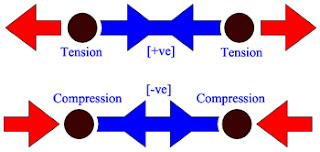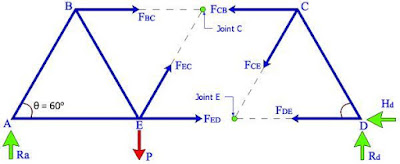# 3 Methods for Truss Analysis

Before discussing the various methods of truss analysis, it would be appropriate to have a brief introduction.

A structure that is composed of a number of bars pin connected at their ends to form a stable framework is called a truss. It is generally assumed that loads and reactions are applied to the truss only at the joints. A truss would typically be composed of triangular elements with the bars on the upper chord under compression and those along the lower chord under tension. Trusses are extensively used for bridges, long span roofs, electric tower, and space structures.

Trusses are statically determinate when the entire bar forces can be determined from the equations of statics alone. Otherwise the truss is statically indeterminate. A truss may be statically (externally) determinate or indeterminate with respect to the reactions (more than 3 or 6 reactions in 2D or 3D problems respectively).Sign convention
For truss analysis, it is assumed that:
• Bars are pin-connected.
• Joints are frictionless hinges.
• Loads are applied at the joints only.
• Stress in each member is constant along its length.
The objective of truss analysis is to determine the reactions and member forces. The methods used for carrying out the analysis with the equations of equilibrium and by considering only parts of the structure through analyzing its free body diagram to solve the unknowns.

### 1. Method of Joints for Truss Analysis

We start by assuming that all members are in tension reaction. A tension member experiences pull forces at both ends of the bar and usually denoted by positive (+ve) sign. When a member is experiencing a push force at both ends, then the bar is said to be in compression mode and designated as negative (-ve) sign.

In the joints method, a virtual cut is made around a joint and the cut portion is isolated as a Free Body Diagram (FBD). Using the equilibrium equations of ∑ Fx = 0 and ∑ Fy = 0, the unknown member forces can be solved. It is assumed that all members are joined together in the form of an ideal pin, and that all forces are in tension (+ve reactions).

An imaginary section may be completely passed around a joint in a truss. The joint has become a free body in equilibrium under the forces applied to it. The equations ∑ H = 0 and ∑ V = 0 may be applied to the joint to determine the unknown forces in members meeting there. It is evident that no more than two unknowns can be determined at a joint with these two equations.

A simple truss model supported by pinned and roller support at its end. Each triangle has the same length, L and it is equilateral where degree of angle, θ is 60° on every angle. The support reactions, Ra and Rc can be determined by taking a point of moment either at point A or point C, whereas Ha = 0 (no other horizontal force).
Here are some simple guidelines for this method:
1. Firstly draw the Free Body Diagram (FBD),
2. Solve the reactions of the given structure,
3. Select a joint with a minimum number of unknown (not more than 2) and analyze it with ∑ Fx = 0 and ∑ Fy = 0,
4. Proceed to the rest of the joints and again concentrating on joints that have very minimal of unknowns,
5. Check member forces at unused joints with ∑ Fx = 0 and ∑ Fy = 0,
6. Tabulate the member forces whether it is in tension (+ve) or compression (-ve) reaction.

The figure showing 3 selected joints, at B, C, and E. The forces in each member can be determined from any joint or point. The best way to start is by selecting the easiest joint like joint C where the reaction Rc is already obtained and with only 2 unknown, forces of FCB and FCD. Both can be evaluated with ∑ Fx = 0 and ∑ Fy = 0 rules. At joint E, there are 3 unknown, forces of FEA, FEB and FED, which may lead to more complex solution compared to 2 unknown values. For checking purposes, joint B is selected to show that the equation of ∑ Fx is equal to ∑ Fy which leads to zero value, ∑ Fx = ∑ Fy = 0. Each member’s condition should be indicated clearly as whether it is in tension (+ve) or in compression (-ve) state.
Trigonometric Functions:
Taking an angle between member x and z…
1. Cos θ = x / z
2. Sin θ = y / z
3. Tan θ = y / x

### 2. Method of Sections for Truss Analysis

The section method is an effective method when the forces in all members of a truss are to be determined. If only a few member forces of a truss are needed, the quickest way to find these forces is by the method of sections. In this method, an imaginary cutting line called a section is drawn through a stable and determinate truss. Thus, a section subdivides the truss into two separate parts. Since the entire truss is in equilibrium, any part of it must also be in equilibrium. Either of the two parts of the truss can be considered and the three equations of equilibrium ∑ Fx = 0, ∑ Fy = 0, and ∑ M = 0 can be applied to solve for member forces.

Using the same model of simple truss, the details would be the same as previous figure with 2 different supports profile. Unlike the joint method, here we only interested in finding the value of forces for member BC, EC, and ED.
Few simple guidelines:
1. Pass a section through a maximum of 3 members of the truss, 1 of which is the desired member where it is dividing the truss into 2 completely separate parts,
2. At 1 part of the truss, take moments about the point (at a joint) where the 2 members intersect and solve for the member force, using ∑ M = 0,
3. Solve the other 2 unknowns by using the equilibrium equation for forces, using ∑ Fx = 0 and ∑ Fy = 0.
Note: The 3 forces cannot be concurrent, or else it cannot be solved.A virtual cut is introduced through the only required members which is along member BC, EC, and ED. Firstly, the support reactions of Ra and Rd should be determined. Again a good judgment is required to solve this problem where the easiest part would be to consider either the left hand side or the right hand side. Taking moment at joint E (virtual point) clockwise for the whole RHS part would be much easier compared to joint C (the LHS part). Then, either joint D or C can be considered as the point of moment, or else using the joint method to find the member forces for FCB, FCE, and FDE. Note: Each value of the member’s condition should be indicate clearly as whether it is in tension (+ve) or in compression (-ve) state.

### 3. Graphical Method of Truss Analysis (Maxwell’s Diagram)

The method of joints could be used as the basis for a graphical analysis of trusses. The graphical analysis was developed by force polygons drawn to scale for each joint, and then the forces in each member were measured from one of these force polygons.The number of lines which have to be drawn can be greatly reduced, however, if the various force polygons are superimposed. The resulting diagram of truss is known as the Maxwell’s Diagram.In order to draw the Maxwell diagram directly, here are the simple guidelines:
1. Solve the reactions at the supports by solving the equations of equilibrium for the entire truss,
2. Move clockwise around the outside of the truss; draw the force polygon to scale for the entire truss,
3. Take each joint in turn (one-by-one), then draw a force polygon by treating successive joints acted upon by only two unknown forces,
4. Measure the magnitude of the force in each member from the diagram,
5. Lastly, note that work proceeded from one end of the truss to another, as this is use for checking of balance and connection to the other end.
A simple triangle truss with degree of angle, θ is 60° on every angle (a equilateral) and same member’s length, L on 2 types of support. Yet again, evaluating the support reaction plays an important role in solving any structural problems. For this case, the value of Hb is zero as it is not influenced by any horizontal forces.The procedure for solving this problem could be quite tricky and requires imagination. It starts by labeling the spaces between the forces and members with an example shown above; reaction Ra and applied force, P labeled as space 1 and continue moving clockwise around the truss. For each member, take example between space 1 and 5 would be the member AC and so forth. Note: Choose a suitable scale for drawing the Maxwell diagram.

In conclusion, the truss internal reactions as well as its member forces could be determined by either of these 3 methods. Nonetheless, the methods of joints becomes the most preferred method when it comes to more complex structures.

3 Methods for Truss Analysis Drop your comments below for any improvements or suggestions. We'll be happy to accommodate your valuable contributions.

3 methods to analyze trusses.

[blogger]

Engineeersdaily

Name

Email *

Message *